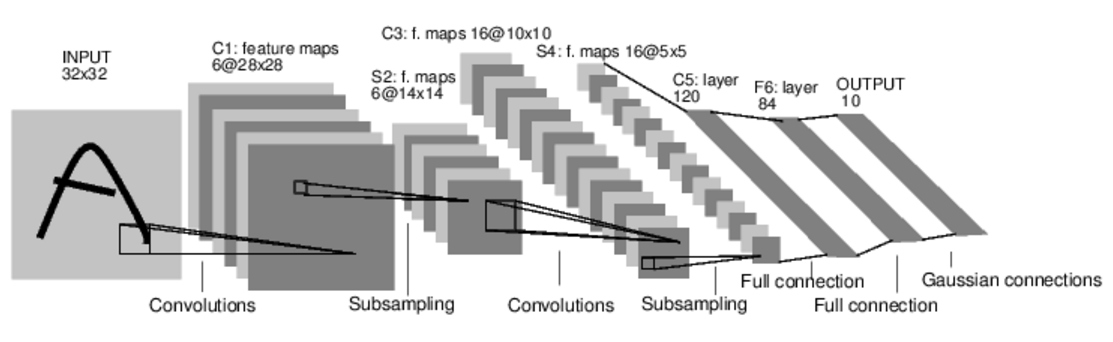# pytorch深度学习60分钟闪电战

2019/03/27 19:23

### Tensors

#Construct a 5x3 matrix, uninitialized:
x = torch.empty(5, 3)

#Construct a randomly initialized matrix:
x = torch.rand(5, 3)

# Construct a matrix filled zeros and of dtype long:
x = torch.zeros(5, 3, dtype=torch.long)

# Construct a tensor directly from data:
x = torch.tensor([5.5, 3])

# create a tensor based on an existing tensor. These methods will reuse properties of the input tensor, e.g. dtype, unless new values are provided by user
x = x.new_ones(5, 3, dtype=torch.double)      # new_* methods take in sizes
print(x)
x = torch.randn_like(x, dtype=torch.float)    # override dtype!    #沿用了x已有的属性,只是修改dtype
print(x)                                      # result has the same size


tensor操作的语法有很多写法,以加法为例

#1
x = x.new_ones(5, 3, dtype=torch.double)
y = torch.rand(5, 3)
print(x + y)

#2

#3
result = torch.empty(5, 3)
print(result)

##注意以_做后缀的方法,都会改变原始的变量
#4 Any operation that mutates a tensor in-place is post-fixed with an _. For example: x.copy_(y), x.t_(), will change x.
print(y)


x = torch.randn(4, 4)
y = x.view(16)
z = x.view(-1, 8)  # the size -1 is inferred from other dimensions
print(x.size(), y.size(), z.size())

torch.Size([4, 4]) torch.Size() torch.Size([2, 8])


numpy array和torch tensor的相互转换

• torch tensor转换为numpy array
a = torch.ones(5)
print(a)

#torch tensor--->numpy array
b = a.numpy()
print(b)

#注意!:a,b同时发生了变化
print(a)
print(b)

[2. 2. 2. 2. 2.]

• numpy array转换为torch tensor
a = np.ones(5)
b = torch.from_numpy(a)


All the Tensors on the CPU except a CharTensor support converting to NumPy and back.

CUDA Tensors

Tensors can be moved onto any device using the .to method.

#let us run this cell only if CUDA is available
#We will use torch.device objects to move tensors in and out of GPU
if torch.cuda.is_available():
device = torch.device("cuda")          # a CUDA device object
y = torch.ones_like(x, device=device)  # directly create a tensor on GPU
x = x.to(device)                       # or just use strings .to("cuda")
z = x + y
print(z)
print(z.to("cpu", torch.double))       # .to can also change dtype together!

--->
tensor([0.6635], device='cuda:0')
tensor([0.6635], dtype=torch.float64)


The autograd package provides automatic differentiation for all operations on Tensors. It is a define-by-run framework, which means that your backprop is defined by how your code is run, and that every single iteration can be different.

Generally speaking, torch.autograd is an engine for computing vector-Jacobian product

torch.Tensor is the central class of the package. If you set its attribute .requires_grad as True, it starts to track all operations on it. When you finish your computation you can call .backward() and have all the gradients computed automatically. The gradient for this tensor will be accumulated into .grad attribute.

### 神经网络

torch.nn包可以用来构建神经网络. nn依赖autogard来不断地更新model中各层的参数. nn.Module包含layers,forward方法.• 定义一个神经网络,含learnable parameters或者叫weights
• 对数据集的所有数据作为Input输入网络
• 计算loss
• 反向传播计算weights的梯度
• 更新weights,一个典型的简单规则:weight = weight - learning_rate * gradient

import torch
import torch.nn as nn
import torch.nn.functional as F

class Net(nn.Module):

def __init__(self):
super(Net, self).__init__()
# 1 input image channel, 6 output channels, 5x5 square convolution
# kernel
self.conv1 = nn.Conv2d(1, 6, 5)    #输入是1个矩阵,输出6个矩阵,filter是5*5矩阵.即卷积层1使用6个filter.
self.conv2 = nn.Conv2d(6, 16, 5)   #输入是6个矩阵,输出16个矩阵,filter是5*5矩阵.即卷积层2使用16个filter.
# an affine operation: y = Wx + b
self.fc1 = nn.Linear(16 * 5 * 5, 120)   #全连接层,fc=fullconnect  作用是分类
self.fc2 = nn.Linear(120, 84)
self.fc3 = nn.Linear(84, 10)

def forward(self, x):
# Max pooling over a (2, 2) window
x = F.max_pool2d(F.relu(self.conv1(x)), (2, 2))
# If the size is a square you can only specify a single number
x = F.max_pool2d(F.relu(self.conv2(x)), 2)
x = x.view(-1, self.num_flat_features(x))
x = F.relu(self.fc1(x))
x = F.relu(self.fc2(x))
x = self.fc3(x)
return x

def num_flat_features(self, x):
size = x.size()[1:]  # all dimensions except the batch dimension
num_features = 1
for s in size:
num_features *= s
return num_features

net = Net()
print(net)

Net(
(conv1): Conv2d(1, 6, kernel_size=(5, 5), stride=(1, 1))
(conv2): Conv2d(6, 16, kernel_size=(5, 5), stride=(1, 1))
(fc1): Linear(in_features=400, out_features=120, bias=True)
(fc2): Linear(in_features=120, out_features=84, bias=True)
(fc3): Linear(in_features=84, out_features=10, bias=True)
)


model的参数获取.

params = list(net.parameters())
print(len(params))
print(params.size())  # conv1's .weight

10
torch.Size([6, 1, 5, 5])


input = torch.randn(1, 1, 32, 32)
out = net(input)
print(out)

tensor([[ 0.0659, -0.0456,  0.1248, -0.1571, -0.0991, -0.0494,  0.0046, -0.0767,

#Zero the gradient buffers of all parameters and backprops with random gradients:
out.backward(torch.randn(1, 10))


• torch.Tensor - A multi-dimensional array with support for autograd operations like backward(). Also holds the gradient w.r.t. the tensor.
• nn.Module - Neural network module. Convenient way of encapsulating parameters, with helpers for moving them to GPU, exporting, loading, etc.
• nn.Parameter - A kind of Tensor, that is automatically registered as a parameter when assigned as an attribute to a Module.
• autograd.Function - Implements forward and backward definitions of an autograd operation. Every Tensor operation creates at least a single Function node that connects to functions that created a Tensor and encodes its history.

#### 损失函数

nn package有好几种损失函数.以nn.MSELoss为例

output = net(input)
target = torch.randn(10)  # a dummy target, for example
target = target.view(1, -1)  # make it the same shape as output
criterion = nn.MSELoss()

loss = criterion(output, target)
print(loss)



Now, if you follow loss in the backward direction, using its .grad_fn attribute, you will see a graph of computations that looks like this:
input -> conv2d -> relu -> maxpool2d -> conv2d -> relu -> maxpool2d -> view -> linear -> relu -> linear -> relu -> linear -> MSELoss -> loss

print(loss.grad_fn)  # MSELoss

<MseLossBackward object at 0x7ff3406e1be0>


#### 反向传播

#首先清空现有的gradient buffer

loss.backward()

tensor([0., 0., 0., 0., 0., 0.])
tensor([-0.0080,  0.0043, -0.0006,  0.0142, -0.0017, -0.0082])


#### 更新权重

learning_rate = 0.01
for f in net.parameters():


import torch.optim as optim

optimizer = optim.SGD(net.parameters(), lr=0.01)

output = net(input)
loss = criterion(output, target)
loss.backward()
optimizer.step()    # Does the update



0
0 收藏

### 作者的其它热门文章0 评论
0 收藏
0